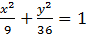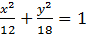### Sample Problem

What is the equation of an ellipse with center at the origin, a vertex at (0,6) and a co – vertex at (3,0)?

(A)(B)(C)(D)#### Solution

Because the vertex is at (0,6), we know that a2 has to be the denominator of y and b2 has to be the denominator or x. Thus, we have